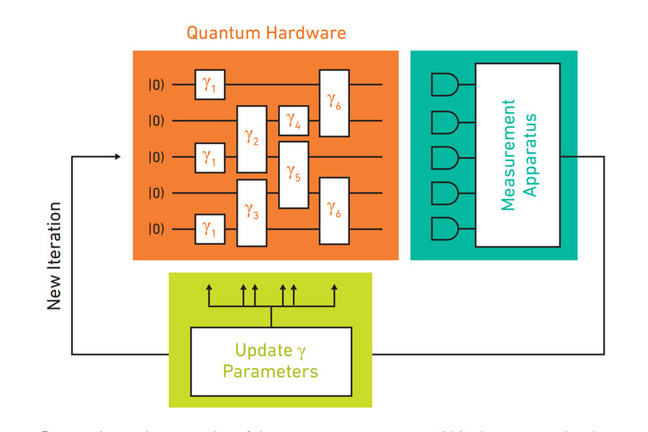3.23

## Keio University" Quantum Information and Computation for Chemistry 2016 " p.10 http://aspuru.chem.harvard.edu/nsf-report/

# Quantum Chemistry

The accurate description of a molecule involves understanding the distance between the atoms and the strength of their atomic bonds (the sharing of electrons so that the atoms stick together in a molecule), the position of the atoms, and the way they vibrate. Much of this work involves understanding what happens as they absorb and release energy, and finding the minimum amount of energy (known as the ground state energy) in the molecule. Answering these questions accurately involves equations with a number of terms that grows exponentially in the precision of the answer you want to get, and is heavily impacted by the number of electrons and atoms involved. This makes the necessary calculations tedious for a classical computer, but ideally suited to a quantum computer.

## The basic problem

The most basic quantum chemistry problem is to find the electronic ground state energy $E_G$ of a molecule, for a particular geometry. Intuitively, the ground state is one that doesn’t change over time, since there is no extra energy in the molecule. This helps chemists to determine the preferred shape of a molecule, which in turn influences how it reacts with other molecules.

To find this energy, we take the mathematical term that represents the global changes to the system over time, called the Hamiltonian of the system (written $H$, but not to be confused with the Hadamard gate we presented last week), and look for a state that doesn’t change as the Hamiltonian is applied. As James Whitfield described in the last Video Step, this process is like knowing that the Earth is spinning but not being certain where the North Pole is, and finding it by searching for a place that doesn’t move as the Earth rotates.

With that state, we can then find the energy, which shows up as a constant factor in the equation. Mathematically, with the state $|\psi\rangle$, the equation is written

$H\vert\psi\rangle = E_G\vert\psi\rangle$.

The vector $|\psi\rangle$ is called the eigenvector and $E_G$ is called the eigenvalue.

In a quantum algorithm, we can read off that $E_G$ when we measure the state. The challenge, then, becomes to prepare the initial state $|\psi\rangle$ and apply the Hamiltonian $H$ efficiently. The most basic approach to solving this problem uses an algorithm called quantum phase estimation.

## Representing data

Our first problem in designing any algorithm is determining what our data representation is going to be. There are a variety of ways we can write down our current condition: either we can keep track of each particle (electron) in the system, or we can keep track of each point in space, for example, like a simulation of weather might do. Which choice is ultimately more efficient depends on the details of the problem.

As Professor Whitfield alluded to, in practice, algorithms may use the qubits to represent a more complex description of the state. In one of the experiments he was involved in, the quantum states were set up to correspond to the relationship between the electrons in the system, rather than the electron position or amplitude at a point. Thus, more qubits gives us a more precise answer to their state relative to each other.

## Hybrid quantum-classical algorithms

Doing the above efficiently is a challenge, and requires that the entire quantum computer be very high fidelity. Illustrated at the top of this article is an alternative approach, iterating between quantum and classical approaches, has been developed. The two most important such algorithms are the variational quantum eigensolver (VQE) and the quantum approximate optimization algorithm (QAOA).

## Problems attacked

Richard Feynman himself performed important work developing algorithms and concepts, and in recent years the teams of Seth Lloyd (MIT), Alan Aspuru-Guzik (Harvard), and Ed Farhi (MIT), and Dave Wecker and the team of Krysta Svore (Microsoft) have worked to make the algorithms concrete. These are far from the only researchers in the area; dozens of researchers assembled at the White House last year for a workshop that produced a report on the field that is very readable and up to date. A link is provided in the Further Study article at the end of this course.

Simple demonstrations that the algorithm works have been done for the hydrogen molecule on several different real quantum computers. In 2017, the IBM Quantum Experience team published a paper demonstrating that their seven-qubit experimental quantum processor (a type of superconducting system; we will learn more about hardware next week) can solve several small problems using these techniques. They worked on molecules as large as beryllium hydride (BeH$_2$). Microsoft has stated that their vision is to better understand a molecule known as FeMoCo, which, as Prof. James Whitfield described in the first week, has the potential to improve the production of agricultural fertilizers.